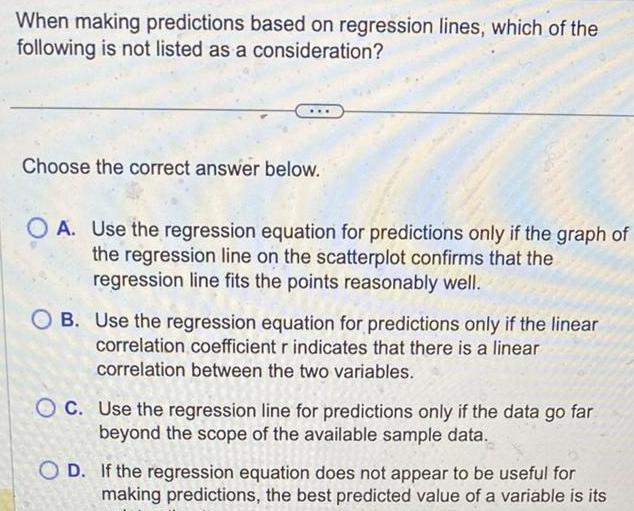Question:

# When making predictions based on regression lines which of

Last updated: 11/20/2023When making predictions based on regression lines which of the following is not listed as a consideration Choose the correct answer below OA Use the regression equation for predictions only if the graph of the regression line on the scatterplot confirms that the regression line fits the points reasonably well OB Use the regression equation for predictions only if the linear correlation coefficient r indicates that there is a linear correlation between the two variables OC Use the regression line for predictions only if the data go far beyond the scope of the available sample data OD If the regression equation does not appear to be useful for making predictions the best predicted value of a variable is its